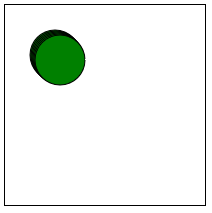### Running functions on an Interval with setInterval - JavaScript basics Tutorial Part 3

Published May 9, 2019, 10:46 p.m.

Welcome to part 3 of the JavaScript basics tutorial series. In this part, we're going to look at a method that will allow us to animate our canvas. To do this, we're going to use the built-in called `setInterval` to run functions based on a programmer-set interval.

Code up to this point:

``````<html>
<body>

<canvas id="myCanvas" width=200px height=200px style="border:1px solid #000000;"></canvas>

<script>
const canvas = document.getElementById('myCanvas');
const context = canvas.getContext('2d');

function blob(x, y, size, color){
context.beginPath();
context.arc(x, y,size,0,2*Math.PI);
context.fillStyle = color;
context.fill();
context.stroke();
}

blob(100,100,25,"green");
blob(25,75,12,"red");
blob(60,22,18,"blue");

</script>
</body>
</html>``````

This produces 3 static "blobs" to the screen, but now I want to give them life. How do we go about making these blobs just move around on their own?

JavaScript has a built-in called `setInterval`. The way this works is you pass the function you want to run on an interval, followed by the number of milliseconds that you want the interval to be. So what we'd like to do is maybe just move the x and y coordinates somehow. To quickly do this, what if we added the following under our constants:

``````            let x = 50;
let y = 50;``````

Then, we can modify our blob function to add one to x and y every time it's called:

``````            let x = 50;
let y = 50;

function blob(x, y, size, color){
context.beginPath();
context.arc(x, y,size,0,2*Math.PI);
context.fillStyle = color;
context.fill();
context.stroke();
x++;
y++;
}``````

Unfortunately, we've got some overlappage, and you need to watch out. As you can see, we're using x, y as our parameters, so this namespace is getting overwritten. Let's modify the function's parameters:

``function blob(x_loc, y_loc, size, color)``

And then again in the arc method, making our full function:

``````            function blob(x_loc, y_loc, size, color){
context.beginPath();
context.arc(x_loc, y_loc,size,0,2*Math.PI);
context.fillStyle = color;
context.fill();
context.stroke();
x++;
y++;
}``````

The ++ bits are used to just increment the variable by 1. You could also do `+=1`, or `x = x+1`, for example.

Now, if we just call this blob function a bunch:

``````            blob(x,y,25,"green");
blob(x,y,25,"green");
blob(x,y,25,"green");
blob(x,y,25,"green");
blob(x,y,25,"green");
blob(x,y,25,"green");``````

Our result:Alright that works. Next, this movement all happened immediately. Instead, we want to slow it down a bit, so that we can see it. To do this, we're going to use the `setInterval` built-in. This takes in a function and amount of milliseconds between running that function, and will just keep running it indefinitely. Let's try that.

The first thing I tried:

``            setInterval(blob(x, y, 25,"green"), 100);``

My line of thinking here is that this would run the blob function every 100 milliseconds. Unfortunately, this seems to result in a static blob and doesn't quite work.

I googled around for what was going on, using the search string: `"modifying variable in a function inside setinterval javascript."` I landed on the following stack overflow answer.

The general issue I found here was that, while `setInterval` will initially use our variable, the values for `x` and `y` will become local to the `setInterval`'s timer function.

Darn! I guess we should just give up.

Just kidding.

While I don't really see how to implement the code from the top answer, I decided to just define the function from within the `setInterval` itself. You can just toss in temporary functions here. I'll refer to this as a throwaway function for now, since that's basically what it is. So what we'll do is basically:

``            setInterval(function(){}, 100);``

Now we just populate that function with what we need. So, I will remove the x++ and y++ from the `blob` function itself, then call the `blob` function, and do the `x++` and `y++` inside our `setInterval`'s `function()`, like this:

``````            setInterval(function(){
blob(x, y, 25,"green")
x++;
y++;
},10)``````

Resulting with:

Alright, the next major fix we need to make is we need to stop the blob from "dragging" across the screen. It should be cleanly drawn anew every time! For this, we just need to clear the canvas. To do this, we reference the context and do a .clearRect. Clear rect does what it sounds like, it will "clear" a rectangle's worth of contents, and we just need to specify the dimensions of the rectangle we want to clear. For now, we'll just clear the entire thing, since that will be super easy. We can do this with:

``context.clearRect(0, 0, canvas.width, canvas.height);``

We're just going to reference the canvas' properties, like the canvas.width and canvas.height. The result now is:

Full code up to this point:

``````<html>
<body>

<canvas id="myCanvas" width=200px height=200px style="border:1px solid #000000;"></canvas>

<script>
const canvas = document.getElementById('myCanvas');
const context = canvas.getContext('2d');

let x = 50;
let y = 50;

function blob(x_loc, y_loc, size, color){
context.clearRect(0, 0, canvas.width, canvas.height);
context.beginPath();
context.arc(x_loc, y_loc,size,0,2*Math.PI);
context.fillStyle = color;
context.fill();
context.stroke();
}

setInterval(function(){
blob(x, y, 25,"green")
x++;
y++;
},10)

</script>
</body>
</html>``````

Alright, what's next? I think we need to impose some limits for this blob. We need to keep it from going off screen. In the next tutorial, we'll be learning about conditionals.

The next tutorial:

• Introduction to programming with JavaScript tutorial

• Functions and more Canvas - JavaScript Basics Part 2

• Running functions on an Interval with setInterval - JavaScript basics Tutorial Part 3
(currently viewing)
• Conditionals - JavaScript basics Tutorial Part 4

• Object Oriented Programming in js - JavaScript Basics Part 5

• Looping with Objects and OOP with JS - JavaScript Basics Part 6